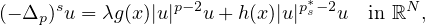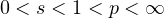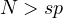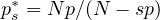Electron. J. Differential Equations, Vol. 2021 (2021), No. 11, pp. 1-17.

### Existence of solutions for critical fractional p-Laplacian equations with indefinite weights Na Cui, Hong-Rui Sun

Abstract:where,and, the weight functions g may be indefinite, and h changes sign. Specifically, based on the results of asymptotic estimates for an extremal in the fractional Sobolev inequality and the discrete spectrum of fractional p-Laplacian operator, we establish an existence criterion for a nontrivial solution to this problem.Na Cui School of Mathematics and Statistics Lanzhou University Lanzhou, Gansu 730000, China email: cuin17@lzu.edu.cn Hong-Rui Sun School of Mathematics and Statistics Lanzhou University Lanzhou, Gansu 730000, China email: hrsun@lzu.edu.cn